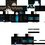Help me!!!

Can you help me the question below?

$\text{Question 1}$

Given that $a+bi=p$ and $a^2-b^2=q$, can you find the value of $ab+i$?

This question is now available in this linkNote by Gia Hoàng Phạm
7 months, 3 weeks ago

This discussion board is a place to discuss our Daily Challenges and the math and science related to those challenges. Explanations are more than just a solution — they should explain the steps and thinking strategies that you used to obtain the solution. Comments should further the discussion of math and science.

When posting on Brilliant:

• Use the emojis to react to an explanation, whether you're congratulating a job well done , or just really confused .
• Ask specific questions about the challenge or the steps in somebody's explanation. Well-posed questions can add a lot to the discussion, but posting "I don't understand!" doesn't help anyone.
• Try to contribute something new to the discussion, whether it is an extension, generalization or other idea related to the challenge.
• Stay on topic — we're all here to learn more about math and science, not to hear about your favorite get-rich-quick scheme or current world events.

MarkdownAppears as
*italics* or _italics_ italics
**bold** or __bold__ bold

- bulleted
- list

• bulleted
• list

1. numbered
2. list

1. numbered
2. list
Note: you must add a full line of space before and after lists for them to show up correctly
paragraph 1

paragraph 2

paragraph 1

paragraph 2

> This is a quote
This is a quote
# I indented these lines
# 4 spaces, and now they show
# up as a code block.

print "hello world"
# I indented these lines
# 4 spaces, and now they show
# up as a code block.

print "hello world"
MathAppears as
Remember to wrap math in $$...$$ or $...$ to ensure proper formatting.
2 \times 3 $2 \times 3$
2^{34} $2^{34}$
a_{i-1} $a_{i-1}$
\frac{2}{3} $\frac{2}{3}$
\sqrt{2} $\sqrt{2}$
\sum_{i=1}^3 $\sum_{i=1}^3$
\sin \theta $\sin \theta$
\boxed{123} $\boxed{123}$

Sort by:

Same solution as @Ram Mohith

$(a+ib)^2=p^2=a^2+i^2b^2+2abi=a^2-b^2+2abi~~~~~\color{#3D99F6}({\text{Since}}~i^2=-1)$$\implies q+2abi~~~~~\color{#3D99F6}({\text{Since the question says that}}~a^2-b^2=q$

$\implies 2abi=p^2-q$

$\implies ab=\frac{p^2-q}{2i}$

$\implies ab+i=\frac{p^2-q}{2i}+i=\frac{p^2-q+2ii}{2i}=\frac{p^2-q-2}{2i}=\frac{-i(p^2-q-2)}{2}=\color{#3D99F6}\boxed{\large{\frac{i(q+2-p^2)}{2}}}$

- 7 months, 3 weeks ago

Unrelated to the problem, but... how do you get that blue text???

- 7 months, 3 weeks ago

I just write \color{blue}\text{blah blah blah ...} and you should get $\color{#3D99F6}\text{blah blah blah}\dots$

- 7 months, 3 weeks ago

$\color{#20A900}\text{Oh I see!}$ First you latex-ify it, and inside you de-latex-ify it using \text{} Clever. Thanks.

- 7 months, 3 weeks ago

\color{blue}\text{Like this?}

- 7 months, 3 weeks ago

I meant backslash left-parenthesis \color{blue}\text{Like this?} backslash right-parenthesis

- 7 months, 3 weeks ago

Hmm, that didn't work.

- 7 months, 3 weeks ago

You should keep them in latex brackets.

- 7 months, 3 weeks ago

Thanks!

- 7 months, 3 weeks ago

Bonus Question

What can you say about the nature of $ab + i$?

\begin{aligned} 1) & \text{ Purely Imaginary} \\ 2) & \text{ Purely Real} \\ 3) & \text{ Complex Number (with both real and Imaginary part)} \\ 4) & \text{ It depends on } p = a + ib \\ \end{aligned}

- 7 months, 3 weeks ago

The answer is option 4

- 7 months, 3 weeks ago

I choose 3) because $a \times i$(purely imaginary) equals to $0+a \times i$ and b(purely real) equals to $a+0 \times i$

Note

• $a$ can be any real number

- 7 months, 3 weeks ago

But are you telling about $ab + i$ or any thing else.

- 7 months, 3 weeks ago

I got the answer as $\boxed{ab + i = \dfrac{q + 2 - p^2}{2} i}$. Here is my solution.

\begin{aligned} (a + ib)^2 & = p^2 \\ a^2 + i^2b^2 + 2abi & = p^2 \\ \underbrace{a^2 - b^2}_{q} + 2abi & = p^2 \qquad (\text{Since } i^2 = -1) \\ ab = & \dfrac{p^2 - q}{2i} \times \dfrac{-i}{-i} \\ ab = & \dfrac{i(q - p^2)}{2} \\ ab + i = & \dfrac{i(q - p^2)}{2} + i \\ ab + i = & \dfrac{q + 2 - p^2}{2} i \\ \end{aligned}

- 7 months, 3 weeks ago

Look closely and you'll see that the real part of p squared is nothing but q.......I think you can proceed from here......

- 7 months, 3 weeks ago